# Resources tagged with: Networks/Graph Theory

Filter by: Content type:
Age range:
Challenge level:

### There are 33 results

Broad Topics > Decision Mathematics and Combinatorics > Networks/Graph Theory### Plum Tree

##### Age 14 to 18 Challenge Level:

Label this plum tree graph to make it totally magic!### Magic Caterpillars

##### Age 14 to 18 Challenge Level:

Label the joints and legs of these graph theory caterpillars so that the vertex sums are all equal.### Olympic Magic

##### Age 14 to 16 Challenge Level:

in how many ways can you place the numbers 1, 2, 3 … 9 in the nine regions of the Olympic Emblem (5 overlapping circles) so that the amount in each ring is the same?### W Mates

##### Age 16 to 18 Challenge Level:

Show there are exactly 12 magic labellings of the Magic W using the numbers 1 to 9. Prove that for every labelling with a magic total T there is a corresponding labelling with a magic total 30-T.### Magic W

##### Age 14 to 16 Challenge Level:

Find all the ways of placing the numbers 1 to 9 on a W shape, with 3 numbers on each leg, so that each set of 3 numbers has the same total.### An Introduction to Computer Programming and Mathematics

##### Age 16 to 18

This article explains the concepts involved in scientific mathematical computing. It will be very useful and interesting to anyone interested in computer programming or mathematics.### Magic W Wrap Up

##### Age 16 to 18 Challenge Level:

Prove that you cannot form a Magic W with a total of 12 or less or with a with a total of 18 or more.### Cube Net

##### Age 16 to 18 Challenge Level:

How many tours visit each vertex of a cube once and only once? How many return to the starting point?### Tangles

##### Age 11 to 16

A personal investigation of Conway's Rational Tangles. What were the interesting questions that needed to be asked, and where did they lead?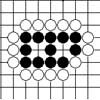### Sufficient but Not Necessary: Two Eyes and Seki in Go

##### Age 14 to 18

The game of go has a simple mechanism. This discussion of the principle of two eyes in go has shown that the game does not depend on equally clear-cut concepts.### Factors and Multiples Graphs

##### Age 16 to 18 Challenge Level:

Explore creating 'factors and multiples' graphs such that no lines joining the numbers cross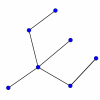### Simply Graphs

##### Age 16 to 18 Challenge Level:

Look for the common features in these graphs. Which graphs belong together?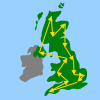### The Olympic Torch Tour

##### Age 14 to 16 Challenge Level:

Imagine you had to plan the tour for the Olympic Torch. Is there an efficient way of choosing the shortest possible route?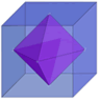### The Four Colour Theorem

##### Age 11 to 16

The Four Colour Conjecture was first stated just over 150 years ago, and finally proved conclusively in 1976. It is an outstanding example of how old ideas can be combined with new discoveries. prove. . . .### Some Circuits in Graph or Network Theory

##### Age 14 to 18

Eulerian and Hamiltonian circuits are defined with some simple examples and a couple of puzzles to illustrate Hamiltonian circuits.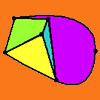### Euler's Formula

##### Age 16 to 18

Some simple ideas about graph theory with a discussion of a proof of Euler's formula relating the numbers of vertces, edges and faces of a graph.### Euler's Formula and Topology

##### Age 16 to 18

Here is a proof of Euler's formula in the plane and on a sphere together with projects to explore cases of the formula for a polygon with holes, for the torus and other solids with holes and the. . . .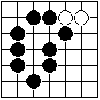### Behind the Rules of Go

##### Age 14 to 18

This article explains the use of the idea of connectedness in networks, in two different ways, to bring into focus the basics of the game of Go, namely capture and territory.### Instant Insanity

##### Age 11 to 18 Challenge Level:

Given the nets of 4 cubes with the faces coloured in 4 colours, build a tower so that on each vertical wall no colour is repeated, that is all 4 colours appear.### Classifying Solids Using Angle Deficiency

##### Age 11 to 16 Challenge Level:

Toni Beardon has chosen this article introducing a rich area for practical exploration and discovery in 3D geometry### Geometry and Gravity 2

##### Age 11 to 18

This is the second of two articles and discusses problems relating to the curvature of space, shortest distances on surfaces, triangulations of surfaces and representation by graphs.### Ding Dong Bell

##### Age 11 to 18

The reader is invited to investigate changes (or permutations) in the ringing of church bells, illustrated by braid diagrams showing the order in which the bells are rung.### Knight Defeated

##### Age 14 to 16 Challenge Level:

The knight's move on a chess board is 2 steps in one direction and one step in the other direction. Prove that a knight cannot visit every square on the board once and only (a tour) on a 2 by n board. . . .### Tree Graphs

##### Age 16 to 18 Challenge Level:

A connected graph is a graph in which we can get from any vertex to any other by travelling along the edges. A tree is a connected graph with no closed circuits (or loops. Prove that every tree has. . . .### Sprouts Explained

##### Age 7 to 18

This article invites you to get familiar with a strategic game called "sprouts". The game is simple enough for younger children to understand, and has also provided experienced mathematicians with. . . .### A Curious Collection of Bridges

##### Age 11 to 18

Read about the problem that tickled Euler's curiosity and led to a new branch of mathematics!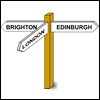### Limiting Probabilities

##### Age 16 to 18 Challenge Level:

Given probabilities of taking paths in a graph from each node, use matrix multiplication to find the probability of going from one vertex to another in 2 stages, or 3, or 4 or even 100.### Symmetric Tangles

##### Age 14 to 16

The tangles created by the twists and turns of the Conway rope trick are surprisingly symmetrical. Here's why!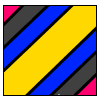### Torus Patterns

##### Age 16 to 18 Challenge Level:

How many different colours would be needed to colour these different patterns on a torus?### Fermat's Poser

##### Age 14 to 16 Challenge Level:

Find the point whose sum of distances from the vertices (corners) of a given triangle is a minimum.### The Use of Mathematics in Computer Games

##### Age 16 to 18

An account of how mathematics is used in computer games including geometry, vectors, transformations, 3D graphics, graph theory and simulations.### Maximum Flow

##### Age 16 to 18 Challenge Level:

Given the graph of a supply network and the maximum capacity for flow in each section find the maximum flow across the network.### Neural Nets

##### Age 16 to 18

Find out some of the mathematics behind neural networks.